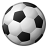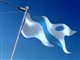# QlikView App Dev

Discussion Board for collaboration related to QlikView App Development.

Announcements
Now Live: Qlik Sense SaaS Simplified Authoring – Analytics Creation for Everyone: READ DETAILS
cancel
Showing results for
Did you mean:Specialist

## If statement -> Loop

Hi all,

I'm using If statement to filter out the data into buckets.

I was wondering whether the same can be achieved by Loop concept.

Any help is highly appreciated.

If(

([Fiscal Week]>=1 and [Fiscal Week]<=4), 1,

If(

([Fiscal Week]>=5 and [Fiscal Week]<=8), 2,

If(

([Fiscal Week]>=9 and [Fiscal Week]<=12), 3,

If(

([Fiscal Week]>=13 and [Fiscal Week]<=16), 4,

If(

([Fiscal Week]>=17 and [Fiscal Week]<=20), 5,

If(

([Fiscal Week]>=21 and [Fiscal Week]<=24), 6,

If(

([Fiscal Week]>=25 and [Fiscal Week]<=28), 7,

If(

([Fiscal Week]>=29 and [Fiscal Week]<=32), 8,

If(

([Fiscal Week]>=33 and [Fiscal Week]<=36), 9,

If(

([Fiscal Week]>=37 and [Fiscal Week]<=40), 10,

If(

([Fiscal Week]>=41 and [Fiscal Week]<=44), 11,

If(

([Fiscal Week]>=48 and [Fiscal Week]<=52), 12)))))))))))) as [Fiscal Month];

6 RepliesMVP

What exactly do you mean when you say loop concept?Specialist

What happens to weeks 45 - 47 ?

Or perhaps they can't exist?Specialist
Author

Hi Richard,

Sorry for the confusion.

Let me re post the question with application atatched.Specialist
Author

Sunny,

Sorry for the confusion.

Let me re post the question with application atatched.MVP & Luminary

Instead of using such a nested if-loop could the use of class(), pick(match()) or a mapping be better alternatives. Whereby I would suggest to create the fiscal month within a fiscal-calendar:

Fiscal Year

Fiscal Calendar with Non-Standard Days (Not 1-31)

- MarcusSpecialist

Its worth noting that the nested IF is processed in sequence, so it could be tidied up as follows and becomes a bit more obvious to anyone trying to understand the script: